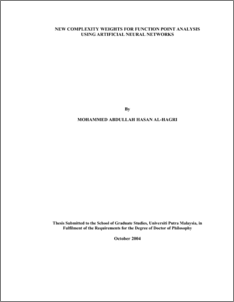# New Complexity Weights for Function Point Analysis Using Artificial Neural Networks

## Citation

Mohammed Abdullah, Hasan Al-Hagri (2004) New Complexity Weights for Function Point Analysis Using Artificial Neural Networks. PhD thesis, Universiti Putra Malaysia.

## Abstract

Function points are intended to measure the amount of functionality in a system as described by a specification. Function points are first proposed in 1979 and currently they are known as the International Function Points User Group (IFPUG) version 4.1. Function points are computed through three steps. The first step is counting the number of the five components in a system which are external inputs, external outputs, external inquiries, external files, and internal files. The second step is assigning a complexity weight to each of the components using weighting factors that are established according to the ordinal scale: simple, average, or complex. The last step is determining 14 technical complexity factors. Although, function points are widely used, they still have limitations. Function points suffer from problem with subjective weighting in the second step since the weights used may not be appropriate. The weights are derived from IBM experience. Besides that, the calculation of function points combines measures from an ordinal scale with counts that are on a ratio scale, thus the linear combinations of the calculation are inconsistent with the measurement theory. As a result, the function points measure used in estimation will produce inaccurate estimates. This thesis proposes new complexity weights for the function points measure by modifying the original complexity weights using artificial neural network algorithm. Particularly the Back Propagation algorithm is employed to derive the proposed complexity weights. The complexity weights derived are established according to an absolute scale which is much more flexible and suitable. The real industrial data sets assembled by the International Software Benchmarking Standard Group are used for comparison between the function point measure obtained using the original complexity weights and proposed complexity weights. The results obtained by proposed complexity weights show improvement in software effort estimation accuracy. The results also show reduction of the error margins in effort estimation where the ratio of average error in using the original complexity weights and the proposed complexity weights is 65% to 35% respectively.Preview
PDF
549767_fsktm_2004_8_abstrak_je__dh_pdf_.pdfView Item